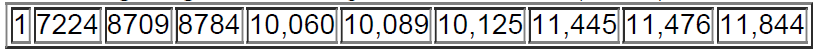×
Get Full Access to Elementary Statistics - 12 Edition - Chapter 3.4 - Problem 29bsc
Get Full Access to Elementary Statistics - 12 Edition - Chapter 3.4 - Problem 29bsc

×

# Boxplots. In Exercises, use the given data to construct aISBN: 9780321836960 18

## Solution for problem 29BSC Chapter 3.4

Elementary Statistics | 12th Edition

• Textbook Solutions
• 2901 Step-by-step solutions solved by professors and subject experts
• Get 24/7 help from StudySoup virtual teaching assistantsElementary Statistics | 12th Edition

4 5 1 393 Reviews
20
3
Problem 29BSC

Boxplots. In Exercises 29–32, use the given data to construct a boxplot and identify the 5-number summary.

Challenger Flights The following are the duration times (minutes) of all missions flown by the space shuttle Challenger.Step-by-Step Solution:

Step 1 of 5

Median=()th value

=()th value

=(5.5)th value

=Average of 5th And 6th value

=(10060+10089)/2

=10074.5

Step 2 of 5

Step 3 of 5

##### ISBN: 9780321836960

This full solution covers the following key subjects: challenger, flown, BSC, boxplots, construct. This expansive textbook survival guide covers 121 chapters, and 3629 solutions. The full step-by-step solution to problem: 29BSC from chapter: 3.4 was answered by , our top Statistics solution expert on 03/15/17, 10:30PM. Elementary Statistics was written by and is associated to the ISBN: 9780321836960. The answer to “?Boxplots. In Exercises 29–32, use the given data to construct a boxplot and identify the 5-number summary.Challenger Flights The following are the duration times (minutes) of all missions flown by the space shuttle Challenger.” is broken down into a number of easy to follow steps, and 34 words. Since the solution to 29BSC from 3.4 chapter was answered, more than 488 students have viewed the full step-by-step answer. This textbook survival guide was created for the textbook: Elementary Statistics, edition: 12.

## Discover and learn what students are asking

Calculus: Early Transcendental Functions : Inverse Trigonometric Functions: Integration
?In Exercises 1-20, find the indefinite integral. $$\int \frac{1}{\sqrt{1-(x+1)^{2}}} d x$$

Calculus: Early Transcendental Functions : Directional Derivatives and Gradients
?In Exercises 13 - 18, find the gradient of the function at the given point. $$w=3 x^{2}-5 y^{2}+2 z^{2}, \quad(1,1,-2)$$

Calculus: Early Transcendental Functions : Second-Order Nonhomogeneous Linear Equations
?Verifying a Solution In Exercises 1-4,verify the solution of the differential equation. Solution

Statistics: Informed Decisions Using Data : Runs Test for Randomness
?What is meant by random? Explain what a run is.

Unlock Textbook Solution